## How to use mathematical concepts and statements

Anybody who has taken an undergraduate mathematics course, and certainly anybody who has taught undergraduate mathematics, will know that there is a huge difference between being familiar with a theorem and knowing how to use it. However, it is a widely adopted convention in textbooks and lectures to give a theorem and its proof and then to hope that the audience will somehow work out how it is applied. One way this is done is through the setting of exercises, and often the main difficulty in solving an exercise is spotting the appropriate theorem to use. Something similar can be true at the research level too: a problem that seems hard to one mathematician may well be easy to another who recognises that it is a consequence of a theorem that is designed to deal with exactly that difficulty.

This is a navigation page with a list of Tricki articles, each of which is entitled "How to use X" for some X. We give the titles and quick descriptions of the articles. (To see the latter, click on the words "Quick description".) Probably it will at some point get too big to be convenient to use. At that point, this page will become a front page with links to more specialized "How to use" pages.

How to use the Baire category theorem

How to use Bayes's theorem

How to use the Bolzano-Weierstrass theorem Quick description ( The Bolzano-Weierstrass theorem asserts that every bounded sequence of real numbers has a convergent subsequence. More generally, it states that if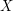is a closed bounded subset of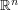then every sequence inhas a subsequence that converges to a point in. This article is not so much about the statement, or its proof, but about how to use it in applications. As explained in the article, there are certain signs to look out for: if you come across one of these signs then the Bolzano-Weierstrass theorem may well be helpful. )

How to use the Cauchy-Schwarz inequality

How to use the central limit theorem

How to use the classification of finite simple groups

How to use cohomology

How to use compactness Quick description ( Compactness is an all-pervasive concept in mathematics. But it is also a tool that can be used for solving problems. This article briefly explains some typical uses and gives links to other more detailed articles.)

How to use the continuum hypothesis Quick description ( Cantor's continuum hypothesis is perhaps the most famous example of a mathematical statement that turned out to be independent of the Zermelo-Fraenkel axioms. What is less well known is that the continuum hypothesis is a useful tool for solving certain sorts of problems in analysis. This article gives a few examples of its use. )

How to use correlation inequalities

How to use duality

How to use entropy

How to use exact sequences

How to use fixed point theorems

How to use the Fourier transform

How to use generating functions

How to use group actions

How to use the Hahn-Banach theorem

How to use the inclusion-exclusion principle

How to use the Lovász local lemma

How to use martingales

How to use the max-flow-min-cut theorem

How to use the mean value theorem

How to use ordinals

How to use the Peirce decomposition Quick description ( If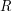is a ring and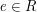is an idempotent, then the Peirce decomposition ofis the decomposition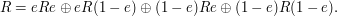)

How to use the pigeonhole principle

How to use the Riemann-Roch theorem

How to use spectral gaps

How to use spectral sequences

How to use Szemerédi's regularity lemma

How to use Talagrand's inequality

How to use tensor products

How to use ultrafilters Quick description ( An ultrafilter on a setis a collection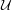of subsets ofwith the following properties: (i)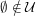; (ii)is closed under finite intersections; (iii) if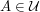and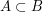then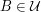; (iv) for every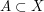, either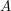or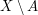belongs to. A trivial example of an ultrafilter is the collection of all sets containing some fixed elementof. Such ultrafilters are called principal. It is not trivial that there are any non-principal ultrafilters, but this can be proved using Zorn's lemma. Here we explain various ways of using non-principal ultrafilters in analysis and infinitary combinatorics. )

How to use Zorn's lemma Quick description ( If you are building a mathematical object in stages and find that (i) you have not finished even after infinitely many stages, and (ii) there seems to be nothing to stop you continuing to build, then Zorn's lemma may well be able to help you.)

The following comments were made inline in the article. You can click on 'view commented text' to see precisely where they were made.

### Apostrophe

Just a note: there seems to be problems with apostrophes. In particular, the `How to use Zorn's lemma' article exists but is listed here as nonexistent.

### Actually the problem was that

Actually the problem was that I'd put a full stop inside the link. I've been discovering quite a few dead-looking links to articles that do in fact exist, and often clicking on the link actually works. It's very helpful to have these pointed out, or just quietly corrected.

## Post new comment

(Note: commenting is not possible on this snapshot.)

Before posting from this form, please consider whether it would be more appropriate to make an inline comment using the Turn commenting on link near the bottom of the window. (Simply click the link, move the cursor over the article, and click on the piece of text on which you want to comment.)